# VLOOKUP CONCATENATE – Excel & Google Sheets

This tutorial will demonstrate how to perform a concatenated VLOOKUP in Excel and Google Sheets.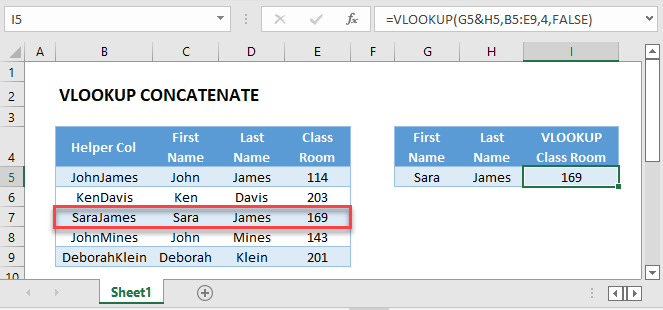## VLOOKUP with Concatenation

You may want to perform a VLOOKUP on a data table without unique values in any single column. In the example below, you want to VLOOKUP on First & Last Name.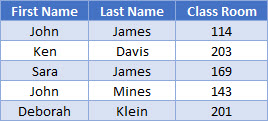In order to do so, you need to create a helper column that merges first and last name together.

``=C3&D3``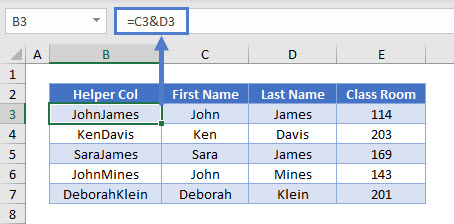In this example, we use the concatenating operator (&), but you could also use the CONCATENATE Function:

``"=CONCATENATE(C3,D3)"``

Once you have the helper column with distinct values, you can perform an exact match VLOOKUP. To get your lookup value for the formula, use the same concatenation as the helper column.

``=VLOOKUP(G3&H3,B3:E7,4,FALSE)``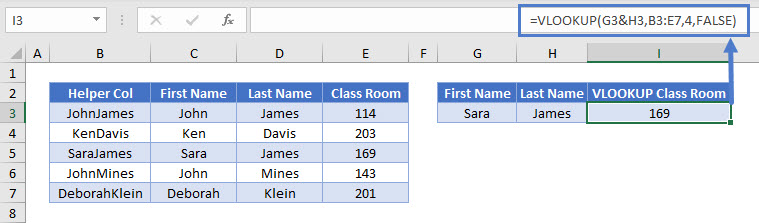## VLOOKUP CONCATENATE in Google Sheets

These formulas work the same in Google Sheets as in Excel.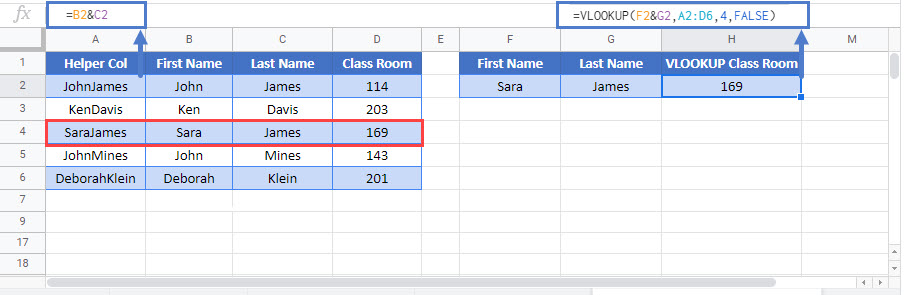### Excel Practice Worksheet

Practice Excel functions and formulas with our 100% free practice worksheets!